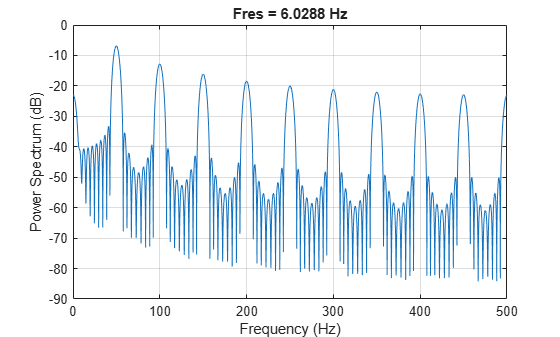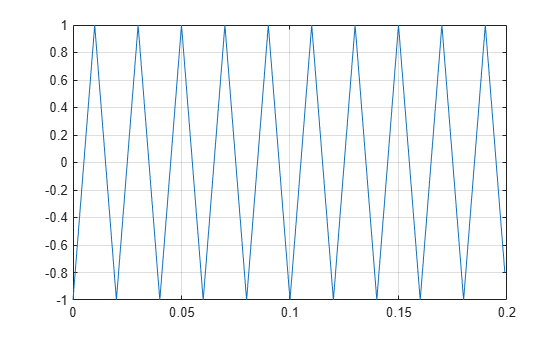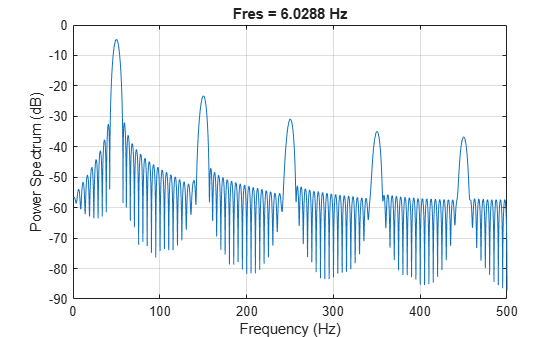# sawtooth

Sawtooth or triangle wave

## Syntax

``x = sawtooth(t)``
``x = sawtooth(t,xmax)``

## Description

example

````x = sawtooth(t)` generates a sawtooth wave with period 2π for the elements of the time array `t`. `sawtooth` is similar to the sine function but creates a sawtooth wave with peaks of –1 and 1. The sawtooth wave is defined to be –1 at multiples of 2π and to increase linearly with time with a slope of 1/π at all other times.```

example

````x = sawtooth(t,xmax)` generates a modified triangle wave with the maximum location at each period controlled by `xmax`. Set `xmax` to `0.5` to generate a standard triangle wave.```

## Examples

collapse all

Generate 10 periods of a sawtooth wave with a fundamental frequency of 50 Hz. The sample rate is 1 kHz.

```T = 10*(1/50); fs = 1000; t = 0:1/fs:T-1/fs; x = sawtooth(2*pi*50*t); plot(t,x) grid on```Plot the power spectrum of the wave.

`pspectrum(x,fs,'Leakage',0.91)`Generate 10 periods of a triangle wave with a fundamental frequency of 50 Hz. The sample rate is 1 kHz.

```T = 10*(1/50); fs = 1000; t = 0:1/fs:T-1/fs; x = sawtooth(2*pi*50*t,1/2); plot(t,x) grid on```Plot the power spectrum of the wave.

`pspectrum(x,fs,'Leakage',0.91)`## Input Arguments

collapse all

Time array, specified as a vector, matrix, or N-D array. `sawtooth` operates along the first array dimension of `t` with size greater than 1.

Data Types: `double`

Wave maximum location, specified as a scalar between 0 and 1. `xmax` determines the point between 0 and 2π at which the wave reaches its maximum. The function increases from –1 to 1 on the interval 0 to 2π × `xmax`, then decreases linearly from 1 to –1 on the interval 2π × `xmax` to 2π. The shape then repeats with a period of 2π.

Example: `xmax = 0.5` specifies a standard triangle wave, symmetric about time π with a peak-to-peak amplitude of 1.

Data Types: `double`

## Output Arguments

collapse all

Sawtooth wave, returned as a vector, matrix, or N-D array.